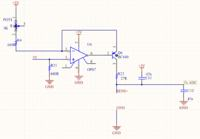# thermometer with pt100 and atmega8

Status
Not open for further replies.

#### mahdiiust

##### Junior Member level 1
Hi
I want to build a thermometer using pt100 and atmega8 microcontroller. First I build a 1mA current source using op07cp and connect it to pt100. Then I read the voltage of pt100 using atmega8 ADC and calculate the resistance. After that by some equations mentioned in pt100 datasheet the temperature will be available (of course in theory!!)
But I encountered a problem. The resistance variation for 1°C increase in temperature is about 390 mΩ. for I mA current and accuracy about 0.1 °C the voltage will be 39 µV. but I can’t read this voltage by atmega8 ADC (Vref=1.2 V, 10 bit). So I try to amplifying it using op amp. Is my scheme true? And which op amp is suitable for amplifying such low voltage? I try Ua741, op07 and LM358 but they didn’t work.
thanksLast edited by a moderator:

Hi,

* at first you have to decide your max. temperature range.
* then calculate min. and max. voltage. According resistance tables, constant_current and min and max temperature.
--> this is the input voltage range of your OPAMP

If i´m correct, then the ADC input voltage range is 0V ... 1.2V
* devide ADC input voltage range by OPAMP input voltage range --> this gives the desired gain of the OPAMP circuit.
* then you need to adjust offset, so that with min. input voltage you get 0V output voltage. (assuming non inverting circuit)
* in the software you need to calculate this back: first you have to correct offset, then adjust gain(best with one multiplication of "Kelvin/LSB")

With a 10 bit ADC you get a temperature resolution of: temperature_range/1024.

Klaus

•mahdiiust

### mahdiiust

Points: 2
The resistance variation for 1°C increase in temperature is about 390 mΩ. for I mA current and accuracy about 0.1 °C the voltage will be 39 µV

You need to calculate the bias current so that the curve fit within the less non-linear region of the sensor. Keep in mind that PT100 sensors are not totally linear. Additionally, you must keep in mind that even the smallest polarization current is able to add a self-heating, sometimes not negligible; therefore you could consider to take the first measurement just after a steady temperature at the probe, particularly at such accuracy.

•mahdiiust

Points: 2